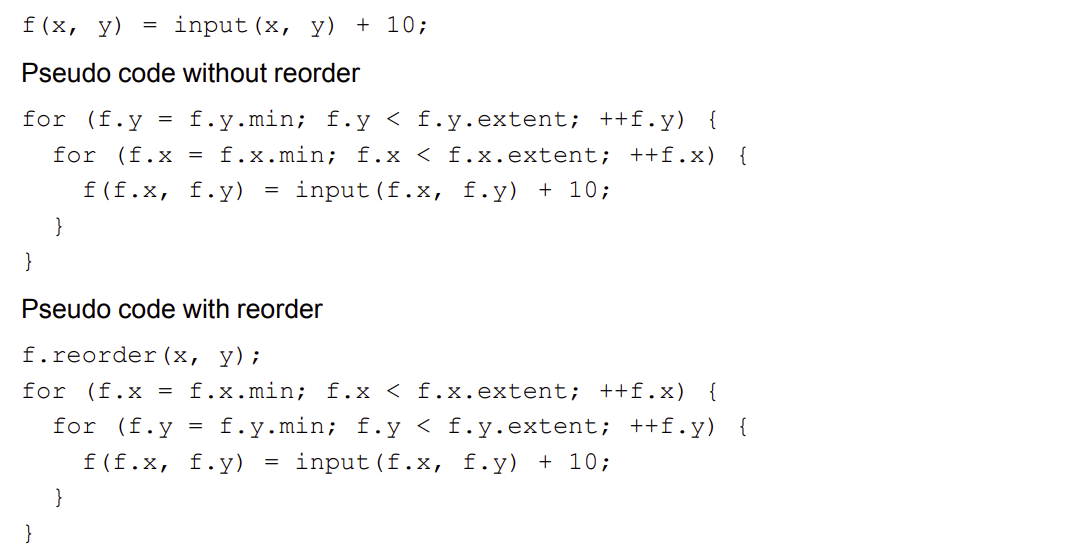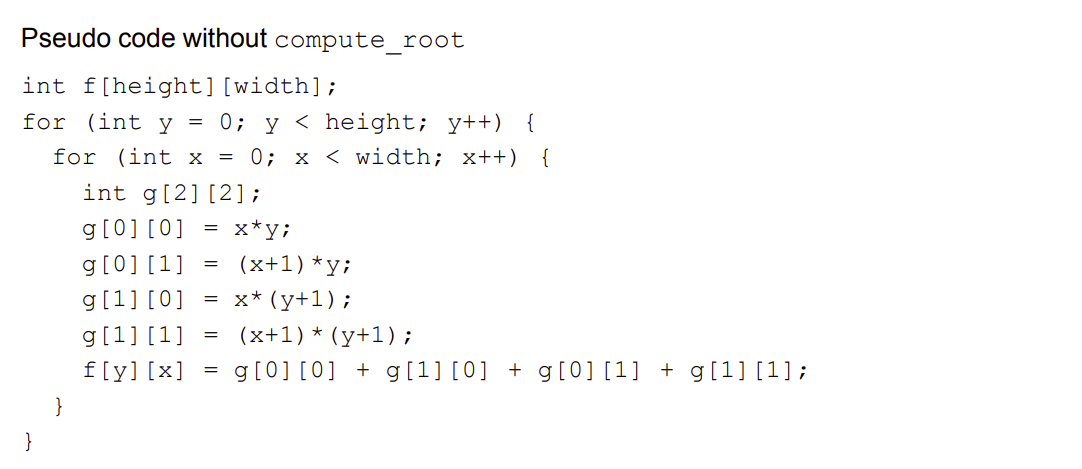## Introduction

Qualcomm has a user guide called Halide for HVX (Hexagon Vector eXtension). The documentation is a great guide to both halide and HVX. I learned many things from the it.

But, besides all the good stuff, I found a couple of mistakes in the documentation which in the beginning confused me. I had to validate some of those to ensure if I had a wrong understanding before. Probably you did too?

1. If someone goes through the same, they can look it up and find some updates here.
2. If Qualcomm Hexagon folks read this doc, they can fix the document and let me know.

In this article I’ll assume you are aware of Halide and Qualcomm’s DSP called Hexagon. Halide has a first class support for generating instructions supported on hexagon called HVX or Hexagon Vector eXtension. If you are not familiar with Halide and are just curious, you can start here.

If you are from Qualcomm and in a position to fix the documentation - please do and let me know. Once again, thanks for the documentation.

Update: I heard back from Qualcomm team, these issues have been addressed. See more context on the update below. I’m keeping this article as is considering its still the document that shows up first in Google search on the topic.

## Issues

Here are a few issues observed.

### Additional update in the example

In page 10, in the description of `Func`.The `input_16` is assigned twice, the 2nd one seems wrong. It should be like this

``````bounded_input(x, y) = BoundaryConditions::repeat_edge(input)(x, y);
input_16(x, y) = cast<int16_t>(bounded_input(x, y));
rows(x, y) = input_16(x, y-2) + 4 * input_16(x, y-1)
+ 6 * input_16(x,y)+ 4 * input_16(x,y+1) + input_16(x,y+2);
// rest of the code.
``````

### Documentation of reorder()

In page 12, in the description of `reorder()` directive.

Here, in the section `Pseudo code with reorder` it says

`f.reorder(x, y);` will generateThis is incorrect. The interface is

``````reorder(dim_inner, .., dim_outer)
``````

Thus `f.reorder(x, y)` will generate opposite of what is shown.

``````f.reorder(x, y);

// Pseudocode with reorder()
for (f.y = f.y.min; f.y < f.y.extent; ++f.y) {
for (f.x = f.x.min; f.x < f.x.extent; ++f.x) {
f(f.x, f.y) = input(f.x, f.y + 10);
}
}
``````

To generate the expected pseudocode (with x in outer loop and y in inner loop), the required schedule is:

``````f.reorder(y, x);
``````

### Documentation of compute_root, compute_at and store_at

In page 17, in the documentation of `compute_root()`

Its documented that, pesudo code without `compute_root()` for

``````Func g("g");
g(x, y) = x*y;
f(x, y) = g(x, y) + g(x, y+1) + g(x+1, y) + g(x+1, y+1);
``````

will generate something likeBut without the explict schedule for intermediate `Func` be computed inline, something like

``````int f[height][width];
for (int y = 0; y < height; y++) {
for (int x = 0; x < width; x++) {
f[y][x] = x*y + (x+1)*y + (x+1)*(y+1) + (x+1)*(y+1);
}
}
``````

Same issue was observed for `store_at()` in page 18 and `store_root` at page 19.

I have a feeling this is intentionally done to make it easier to understand. Even if this is the case - it’s inaccurate.

• `4th May 2022` I have sent emails to `support.cdmatech@qti.qualcomm.com` / `halide@quicinc.com.` (reference from the document). I’ll update this article in case there is some updates or response.
• `5th May 2022` I heard back from the lead from the Halide team at Qualcomm. Here’s the summary from the email: